# Percent of a Number Equations Lesson Plan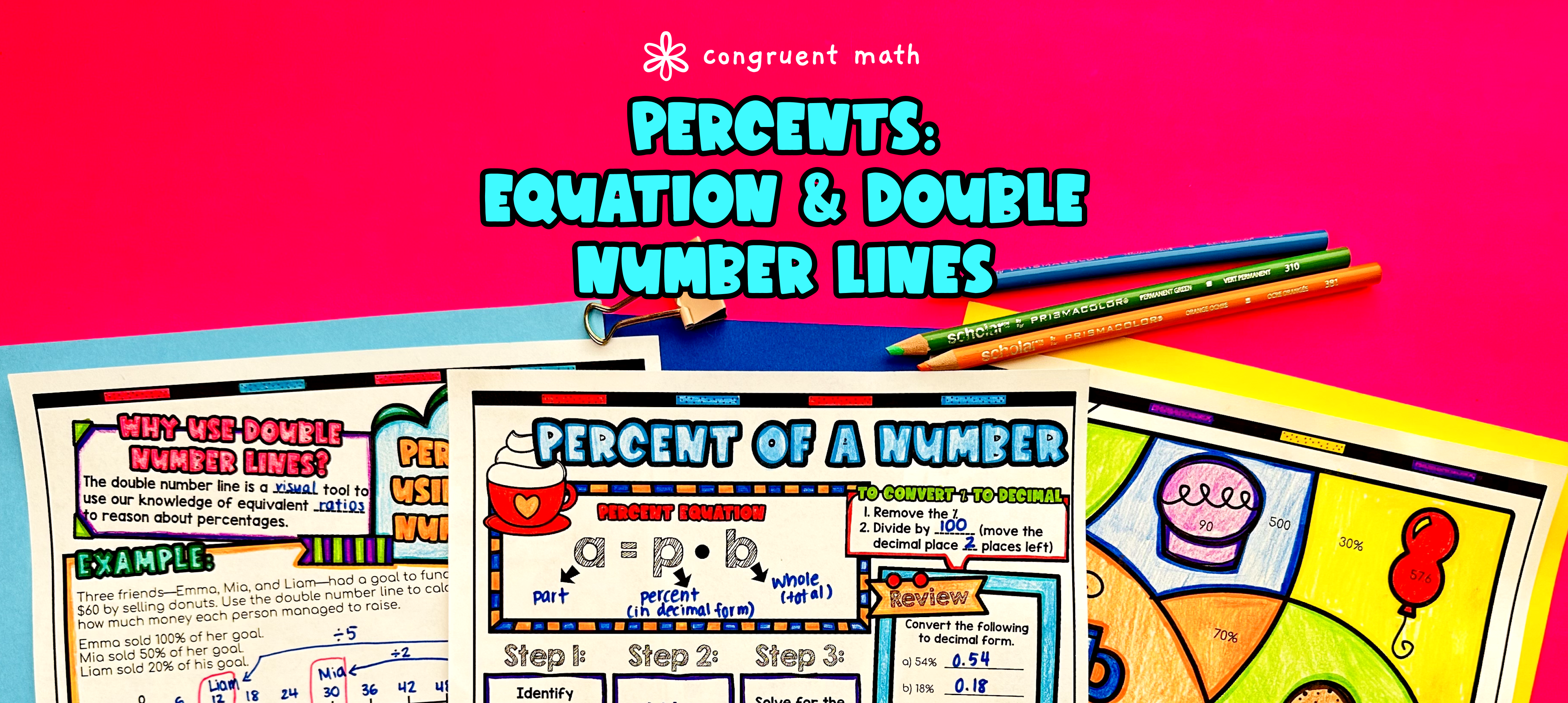Pin This

## Overview

Ever wondered how to teach percent of a number equations in an engaging way to your eighth-grade students?

In this lesson plan, students will learn about finding the part, whole, and percent using percent equations and double number lines. Through artistic and interactive guided notes, check for understanding, a doodle and color by number activity, and a maze worksheet, students will gain a comprehensive understanding of percent equations and double number lines.

The lesson culminates with a real-life example that explores the real-life applications of percentages in word problems. Students will have the opportunity to apply their knowledge and see how percentages are used in practical situations.

## Get the Lesson Materials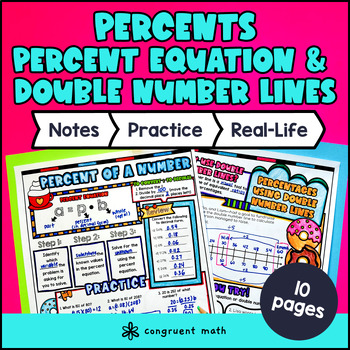\$4.25

## Learning Objectives

After this lesson, students will be able to:

• Define what a percentage is and identify its relationship to a part, whole, and percent

• Use the percent equation to find the part, whole, or percent when given any two of the three values

• Solve word problems involving percentages using the percent equation

• Understand how to use a double number line to represent and solve percentage problems

• Explain real life applications for calculating percent of a number

## Prerequisites

Before this lesson, students should be familiar with:

• Basic knowledge of decimal operations

• Ability to solve simple equations (one step equations)

## Key Vocabulary

• Percent

• Part

• Whole

• Percent equation

• Double number line

## Procedure

### Introduction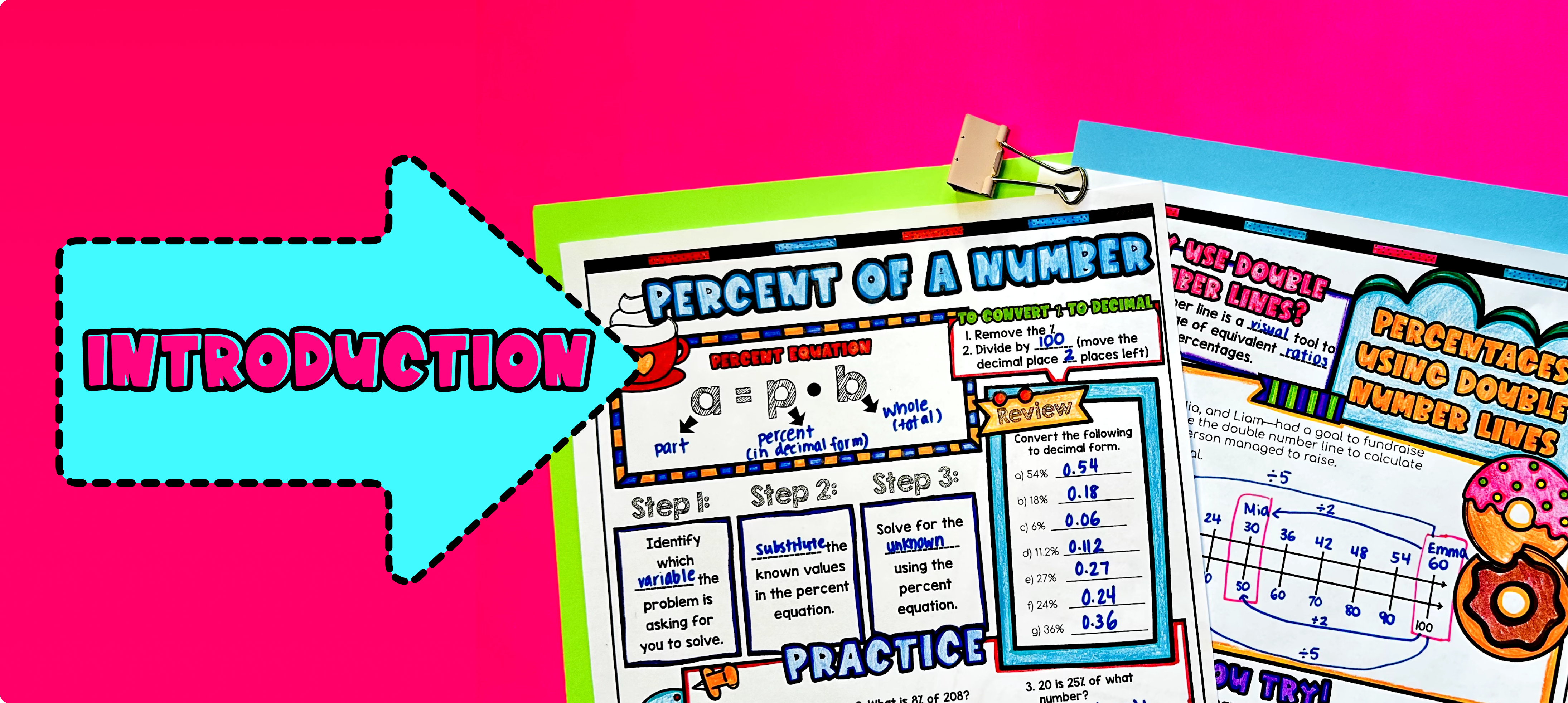Pin This

As a hook, ask students why learning about percentages is important in real life. You can give examples such as calculating discounts while shopping, finding the tip for a meal at a restaurant, or understanding interest rates on loans or savings accounts. Refer to the last page of the guided notes as well as the FAQs below for more ideas.

Use the first page of the guided notes to introduce the concept of finding the part, whole, and percent using the percent equation. Then, use the second page of the guided notes to showcase how to represent and solve percent problems using a double number line. Students will then get to choose which methods they prefer to use for the rest of the practice.

Based on student responses, reteach any concepts that students may need extra help with. If your class has a wide range of proficiency levels, you can pull out students for reteaching, and have more advanced students begin work on the practice exercises.

### Practice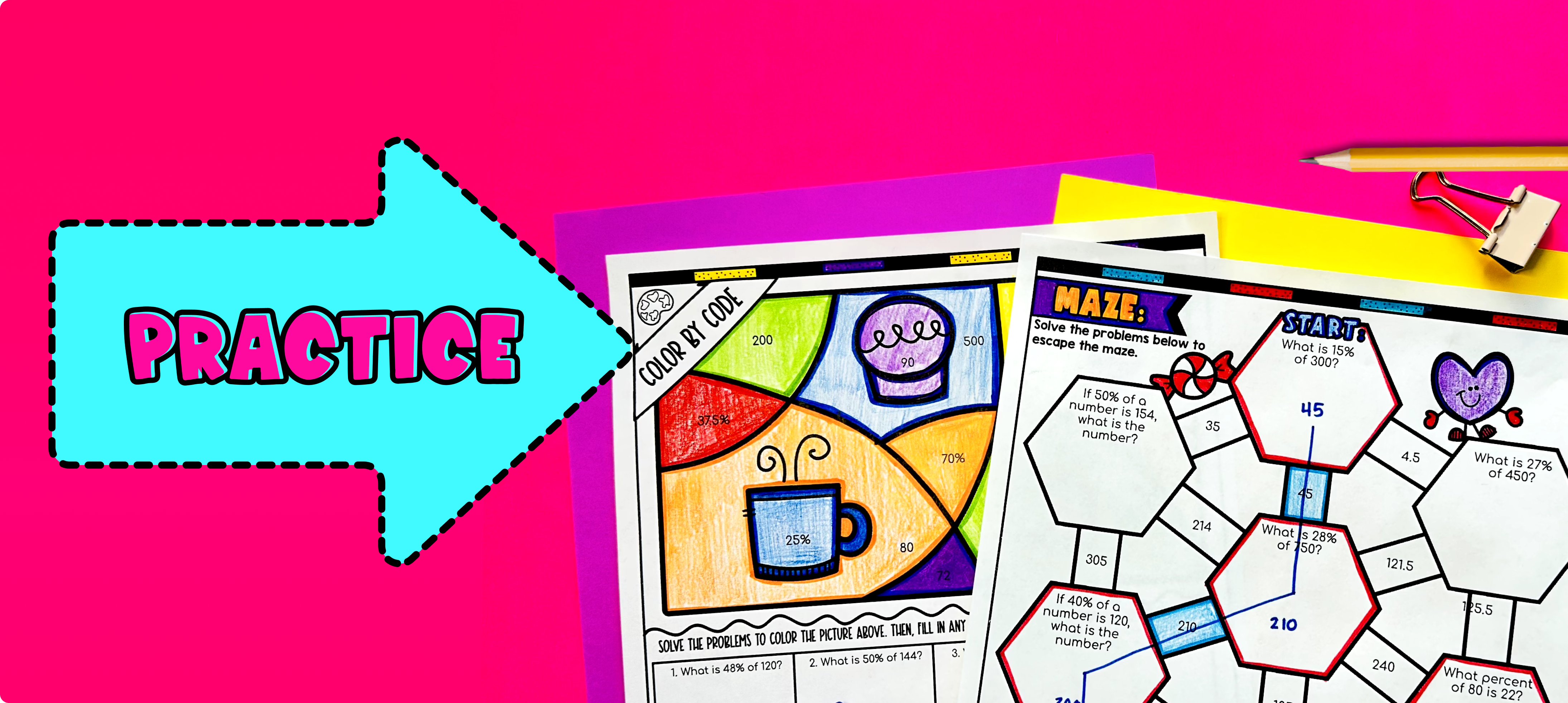Pin This

Have students practice finding the part, whole, and percent using the percent equations and double number lines using the practice activities (maze + color by number worksheets within the guided notes).

Walk around the classroom to answer any student questions and provide clarification as needed. You can also assign the practice worksheet as homework to allow students to continue practicing and reviewing the concepts covered in class.

### Real-Life Application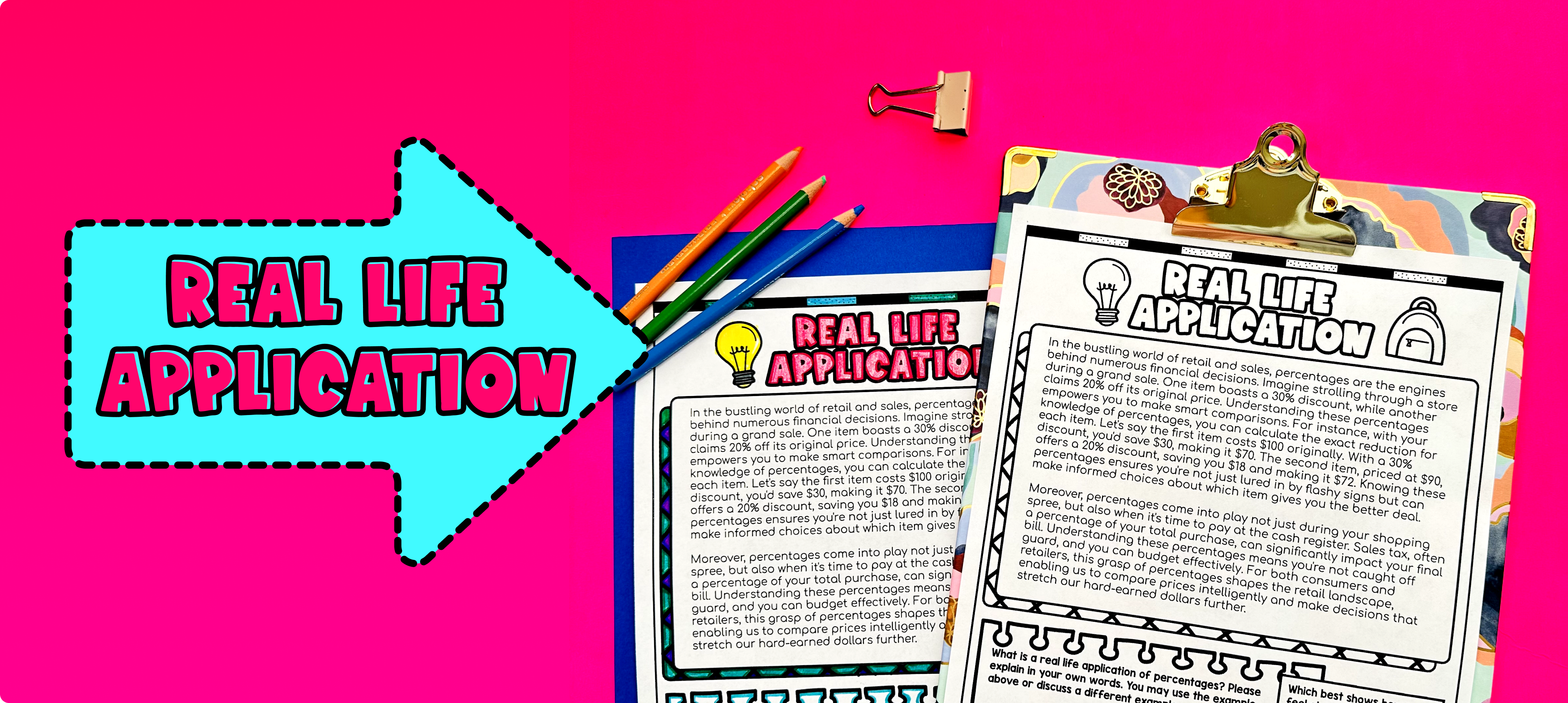Pin This

Bring the class back together, and introduce the concept of real-world applications of finding the part, whole, and percents. Use the last page of the guided notes to provide students with a detailed real life application of percentages. Explain that percentages are used in many different areas of our lives, such as shopping, finance, and statistics.

To help students understand the real-life application of percentages, refer to the FAQ section of the resource for more ideas on how to teach it. You can also provide examples or scenarios for students to analyze and solve using the skills they have learned in the guided notes. Encourage students to think critically and apply their knowledge to practical situations.

## Extensions

### Doodle Math Worksheets

A fun, no-prep way to practice finding the part, whole, and percent using percent equations and double number lines is Doodle Math — they’re a fresh take on color by number or color by code. It includes multiple levels of practice, perfect for a review day or sub plan.

Here are a few activities to try:

### Real-Life Math Project

A fun way to wrap this lesson with your students is with one of the real-life math projects. In this FREE project, students play the role of an airline intern, using percentages to solve multiple problems. They enable students to see the application of the math in an engaging, extended project: